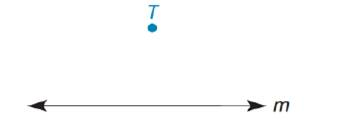Chapter 2.1, Problem 38EElementary Geometry For College St...

7th Edition
Alexander + 2 others
ISBN: 9781337614085

Solutions

Chapter
SectionElementary Geometry For College St...

7th Edition
Alexander + 2 others
ISBN: 9781337614085
Textbook Problem

Given: A line m and a point T not on mSuppose that you do the following:i) Construct a perpendicular line r from T to line m .ii) Construct a line s perpendicular to line r at point T .What is the relationship between lines s and m ?

To determine

To construct:

A perpendicular line r from T to line m and a line s perpendicular to line r at point T. Find the relationship between lines s and m.

Explanation

Approach:

Draw a straight line r from T in such a way that it is perpendicular to m.

Figure (1)

The line r from point T is perpendicular to line m is shown in figure (1).

Construct a straight line from point T in such a way that it is perpendicular to line r.

Still sussing out bartleby?

Check out a sample textbook solution.

See a sample solution

The Solution to Your Study Problems

Bartleby provides explanations to thousands of textbook problems written by our experts, many with advanced degrees!

Get Started

Find more solutions based on key concepts